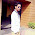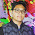# 7 Maths Problem For \$7 Million Give A Try

## 7 Maths Problem For \$7 Million Give A Try

Do you want to be a millionaire? then you are at the right place.If you are good in mathematics then you can make  \$7 million Oh!!, sorry \$6 million one of the question was solved at 2002 by a Russian Mathematician named Grigori Perelman who solved 100 years old maths problem. He proved the Poincare Conjecture. And now there are only 6 unsolved problems left for you to solve and try.

Each of them is for \$1 Million and they are the most difficult problem of mathematics.
We can`t help but be intrigued by the unsolved mysteries. The millennium problem was first laid down by Clay Mathematic Institute in 2000.

So, here is the Seven-millennium problem.

1. P vs NP Problem
2.Riemann Hypothesis
3.Yang- Mills and Mass Gap
4.Navier-Stroke Equation
5.Hodge Conjecture
6.Birch and Swinnerton - Dyer Conjecture.
7.Poincare Conjecture

As mentioned above one of the seven problems was solved by a Russian mathematician he proved Poincare Conjecture which has stumped mathematics since 1904. Grigori turned down the \$1 million prize. In 2002 Perelman also refused the Field Medal, which is the highest honor in mathematics. No one knows the reason why he refused. You`re probably wondering why, well that is another unanswered question for you to Solve.

However, all the above question you read one was P vs NP which is considered the most notorious problem in the world of Computer Science. It`s notorious since it remains an unsolved problem. It basically asks this:
If it is easy to check that a solution to a problem is correct, is it also easy to solve that problem?
Don't think that  I have the answer come back when you have the answer.

Why is it so hard?

In the P vs NP problem, the P stand for Polynomial and NP stand for Nondeterministic Polynomial time. Are you still reading? OK, the in simple term P stand for problems which are easy for computers to solve and NP stand for Problems that are not easy for computers to solve but are easy for them to check.

Here`s an example:

A farmer wants to take 100 watermelons to the market. He needs to pack the watermelons into the boxes. Each box can hold 20 kilograms without breaking. The farmer needs to know if 10 boxes will be enough for him to carry all 100 watermelons to market.
to solve this you have to go through several possible combinations that won't be easy at all. However checking the final answer is pretty easy, Isn't it?  All P problems are also NP problems, (if the computer can easily solve it, the computer can also easily check it). An open challenge to you: Are P problems and NP problems the same type of problems? Or are there are some problems that are easily verified but not easy to solve?

IMG source: Wikipedia

So, Now Tell Me Who Want To Become A Millionaire?

I know that you are thinking who cares about this sort of things. Well if someone could show P = NP then it would make solving difficult real life problems for computer a piece of cake. Oh, and the person will get \$1 million from Clay Mathematics Institute for sure.  Go and give a try.

#### 2 comments :

1.Nice one... Partially usfull

2.THanks for the compliment.